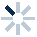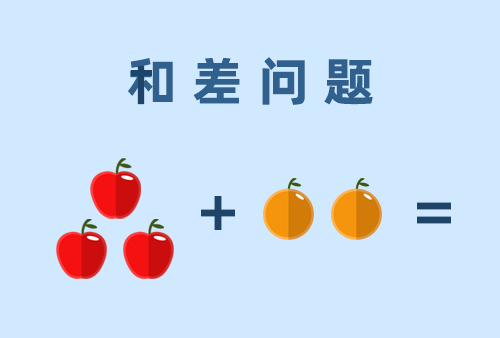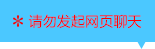| | |

||
APP端下载AndroidiPhone
|
￥35

|
3人点赞
154人已学习
|

3天无理由退款

3天无理由退款：退款将以超级币形式退至您的超级课堂学习账户，便于您重新选购其他课程。恶意退款将被冻结账号。

• 1、概率用来精确描述事件发生的可能性。事件发生的可能性越大，概率就越大；可能性越小，概率就越小
2、 必然事件的概率为$1$，不可能事件的概率为$0$，随机事件的概率就介于$0$到$1$之间
3、 把基本结果满足有限性和等可能性的试验称为古典概率模型，简称为古典概型
4、 事件$A$的概率为$P(A)=\dfrac{m}{n}$。$n$为试验的可能结果数，$m$为事件的可能结果数。这个概率公式也有两种变形，也经常使用
• 1、$m$、$n$一般有三种求法：枚举法、排列组合法与排除法。
2、 枚举法就是把试验的可能结果或事件的可能结果一一列出来，从而求出$m$或$n$。
3、 排列组合法就是用排列组合公式来求$m$、$n$。
4、 若排列组合数不好求，不妨用排除法来间接求。
• 1、我们区分了频率和概率这两个概念。
2、 频率是试验进行完毕后统计出来的结果，不同试验得到的结果可能不同，具有波动性的。
3、 而概率是理论计算出来的，只要试验条件不变就不变。在试验的次数较少时，频率可能和概率会有很大的偏差。但随着试验次数的增多，频率会逐渐接近概率。
• 1、几何概型的含义：如果每个事件发生的概率只与构成该事件区域的长度（面积或体积）成比例，则称这样的概率模型为几何概型
2、 几何概型的特点为无限性与等可能性。其中无限性是几何概型与古典概型的区别
3、 掌握几何概型中概率的公式。而求几何概型的概率，关键就是借助相关的几何图形的长度、面积或体积的比值来求解
• 1、​介绍两类相关性事件及其概率求法：①相互独立事件：如果事件$A$是否发生对事件$B$发生的概率没有影响，这样的两个事件叫做相互独立事件。如果事件$A$和$B$为相互独立事件，那么$A$和$B$都发生的概率等于事件$A$与事件$B$发生的概率之积，即$P(A)×P(B)$。这个结论也可以看作概率中的乘法原理。推广结论为：如果$A_{1}$、$A_{2}$、…，$A_{n}$相互独立，那么它们都发生的概率等于$P(A_{1})×P(A_{2})×\cdots ×P(A_{n})$
2、 ②互斥事件：如果事件$A$与事件$B$不能同时发生，那么这样的两个事件叫做互斥事件。如果事件$A$和$B$为互斥事件，那么$A$和$B$中有一个发生的概率（$A$或$B$发生的概率）等于事件$A$与事件$B$发生的概率之和，即$P(A)+P(B)$。这个结论也可以看作概率中的加法原理。推广结论为：如果$A_{1}$、$A_{2}$、…，$A_{n}$互斥，那么它们当中有一个发生的概率等于$P(A_{1})+P(A_{2})+\cdots +P(A_{n})$
• 1、如果两个事件在任何一次试验中有且仅有一个发生，那么这两个事件就叫做对立事件
2、 如果事件$A$和$B$为对立事件，那么$P(A)+P(B)=1$，即$P(A) =1- P(B)$。这个结论可以看作概率中的排除法
3、 排除法常常用来解决用“至多”、“至少”来限定的问题：先求它的对立事件的概率，再用$1$和它作差，这样可以减少很多分类的麻烦
• 1、含义：独立重复试验是在同样的条件下重复地、相互独立地进行的一种随机试验，它有两大特征：独立与双结果
2、 推导在$n$次独立重复试验中，事件$A$恰好发生$k$次的概率：$C^{k}_{n}×P^{k}×(1-P)^{n-k}$。实际计算时只需分两步：先组合，再和每次试验对应的概率相乘
3、 将多人试验转化为一人独立重复试验的技巧是，用在每个人在做同一个试验时两种结果的概率相同的情况
• 综合练习

• 1超级学员990841
• 2一念之间
• 3超级学员3822887
• 4超级学员3868679
• 5超级学员4214964
• 6一班曹致晟
• 7柯豫我老婆
• 8超级学员4237451
• 9超级学员4238391226395人在学
￥ 16 ￥ 16223812人在学
￥ 28 ￥ 28218432人在学
￥ 32 ￥ 32287787人在学
￥ 0 ￥ 0

• 0
• 点击分享有好礼
••app端下载关注微信号